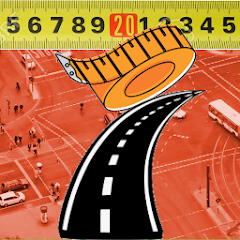# Setting out-Tape and Offset Me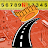100+Everyone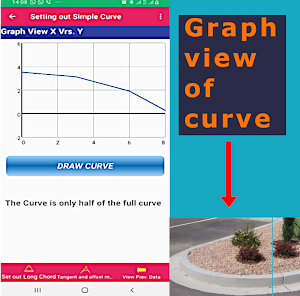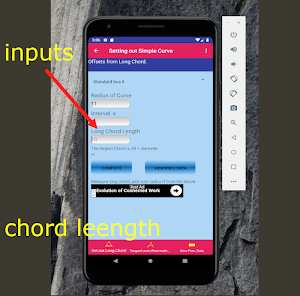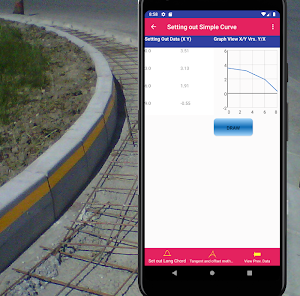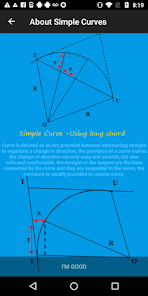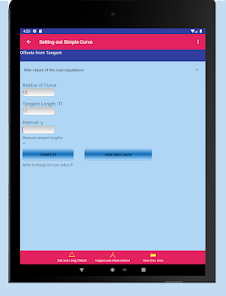There are 3 methods by which pegs on the centreline of circular curves can be set out:1.Tangential angle method2.

Offsets from the tangent lengths 3.
Offsets from the long chordWhen traditional methods are being used it is first necessary to locate the intersection and tangent points of a curve. This procedure is carried out as follows:

Locate the two straights AC and BD and define them with at least two pegs on the ground for each straight. Use nails in the tops of the pegs to define them precisely.Set up a theodolite over the nail in a peg on one of the straights (say AC) and sight the nail in another peg on AC so that the theodolite is pointing in the direction of intersection point IDrive two additional pegs x and y on AC such that straight BD will intersect the line xy. Again use nails in x and y for precisionJoin the nails in the tops of pegs x and y using a string line.Move the theodolite and set it up over a peg on BD, then sight the other peg on BD so that the telescope is again pointing at I.Fix the position of I by driving a peg where the line of sight from the theodolite on BD intersects the string line xy.Move the theodolite to I and measure the angle AIB. Calculate the deflection angle θ, from θ = 180o– angle AIB.

Calculate the tangent lengths IT and IU using [R tan (θ/2)]. Fix points T and U by measuring back along the straights from I.Check the setting out angle ITU which should equal to (θ/2).Locating I, T and U with two InstrumentsIn some cases it may not be possible to locate a theodolite on point I due to inaccessibility of some kind. In this case the location of I and the tangent points can be carried out using two theodolites. The procedure in this case is as follows:

Choose two points A and B somewhere on the straights such that it is possible to sight A to B and B to A and also to measure AB.Measure ABMeasure the angles α and β, calculate λ from λ = 180 – (α + β) and obtain θ from θ = (α + β).Use the sine rule to calculate IA and IB Calculate the tangent lengths IT and IU using [R tan (θ/2)].Using AT = IA – IT and BU = IB – IU set out T from A and U from B. If possible check that ITU is equal to (θ/2)

Setting Out Using Tangential AnglesThis is the most accurate method of the traditional methods for setting out curves. It can be done using a theodolite and tape, two theodolites or a total station and pole reflector.The formula used to determine the tangential angles is derived as follows:

Using a Theodolite and tapeIn this method a theodolite is set up at the tangent point and used to turn the tangential angles to define the directions to each centre line peg. The exact positions of these pegs are fixed by measuring with a tape from peg to peg in sequence.

The exact calculation and setting out procedures are as follows:Setting out Procedure:Using the methods described previously, the tangent points are fixed and the theodolite is set up at one of them.
Updated on
Nov 3, 2020

## Data safety